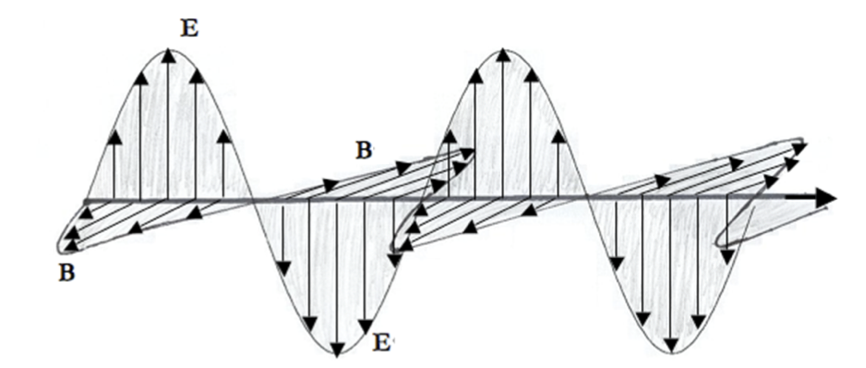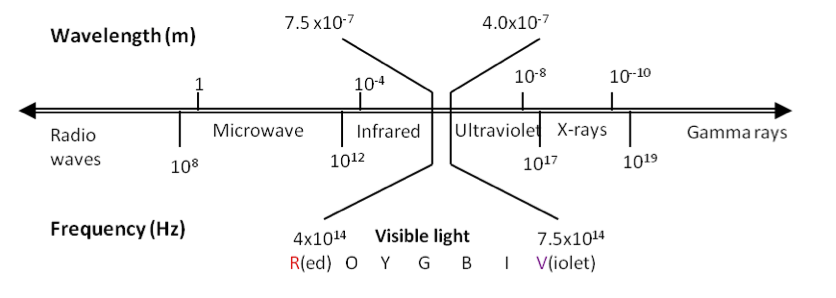## Section46.1Spectrum and Photons

Classically light is an electromagnetic wave consisting of electric and magnetic field waves. According to the Maxwell's equations of electricity and magnetism, oscillating electric field produces oscillating magnetic field and oscillating magnetic field produces oscilalting electric field. This makes the propagation of electromagnetic waves self-sustaining and does not require a medium to propagate. That is why light can reach us from far away stars with vacuum in empty space.

The electric and magnetic fields of an electromagnetic (EM) wave in vacuum are perpendicular to each other and perpendicular to the direction of the wave motion as shown in Figure 46.1.1. These waves are also called transverse electromagnetic waves since the electric and magnetic fields oscillate in a plane perpendicular to the direction of the travel of the wave.Figure 46.1.1. Plane electromagnetic wave traveling to the right. The electric field and magnetic field are perpendicular to each other and direction of the wave is perpendicular to both electric and magnetic fields.

Only a small fraction of frequencies of EM waves is visible to the human eye. The frequency distribution of EM waves is called EM spectrium. Different ranges of frequencies of EM spectrum are given their own name as shown in Figure 46.1.2. Often the visible range of the electromagnetic spectrum is simply called light. Visible light spectrum is further subdivided into colors, which are red, orange, yellow, green, blue, indigo, violet, in order of increasing frequencies or in order of decreasing wavelengths.Figure 46.1.2. The electromagnetic spectrum. The wavelengths given are for EM waves in the vacuum, where EM wavespeed is $3.0\times 10^{8}\text{ m/s}\text{.}$ In other media, the wave speed would be different and hence wavelength for the same frequency wave will be different.

Recall frequency $f\text{,}$ wavelength $\lambda$ and speed $v$ of a wave are related by a fundamental equation for waves.

\begin{equation} f\lambda = v.\tag{46.1.1} \end{equation}

Therefore, higher frequency will correspond to smaller wavelength. Visible light ranges from a frequency of $4\times 10^{14}\text{ Hz}$ to $7.5\times 10^{14}\text{ Hz}\text{,}$ which corresponds to the wavelength range from $7.5\times 10^{-7}\text{ m}$ to $4.0\times 10^{-7}\text{ m}\text{.}$

In the year 1900, Max Planck of Germany hypothesized that the energy contained in the electromagnetic waves must be an integral multiple of a fundamental unit of energy for light of that frequency. For light of frequency $f\text{,}$ the fundamental unit of energy, to be denoted by $E_\gamma$ is

\begin{equation} E_\gamma = h f,\tag{46.1.2} \end{equation}

where $h$ is a universal constant with value

\begin{equation} h = 6.626\times 10^{-34}\text{ J.s}.\tag{46.1.3} \end{equation}

According to Planck, the energy $E$ in a beam of light wave of frequency $f$ is given by

\begin{equation} E = N h f,\tag{46.1.4} \end{equation}

for some integer value for $N\text{.}$

In the year 1905, known as Einstein's year of miracles (annus mirabilis), Albert Einstein extended Planck's idea and reintroduced the particle picture of light. These particles of light, called cospuscles by Sir Isaac Newton, are now called photons. Using letter $c$ for speed of light, the energy $E_\gamma$ and momentum $p_\gamma$of each photon is given by

\begin{equation} \text{Photon particles: }\ \ E_\gamma = hf,\ \ p_\gamma=\frac{E_\gamma}{c} = \frac{hf}{c}\tag{46.1.5} \end{equation}

Since $h$ is a small number in SI units, one photon has very a small amount of energy. Consequently, there are enormous number of photons in any reasonably macroscopic amount of energy. For instance, a $3\text{-mW}$ He-Ne red laser of frequency $4.74 \times 10^{14}\text{ Hz}$ emits approximately $10^{16}$ photons per second as the following calculation shows.

\begin{align*} N \amp = \frac{\text{Power}}{\text{Energy of one photon}} = \frac{P}{hf} \\ \amp = \frac{3\times 10^{-3}\ \text{J/s}}{6.626\times 10^{-34}\ \text{J.s} \times 4.74 \times 10^{14}\ \text{Hz}} = 9.6\times 10^{15}\ \text{per sec}. \end{align*}

Due to the enormity of the number of photons in ordinary light, we do not perceive the cospuscular nature of light. Instead, light appears to us as a continuous stream of energy, much like water in a river.

Photons also carry momentum. For instance, momentum carried by one photon of the red laser light of He-Ne laser is

\begin{align*} p_\gamma \amp = \frac{E_\gamma}{c} = \frac{hf}{c} \\ \amp = \frac{6.626\times 10^{-34}\ \text{J.s} \times 4.74 \times 10^{14}\ \text{Hz}}{3.0\times 10^{8}\text{ m/s}} = 1.05 \times 10^{-27}\text{ N.s}. \end{align*}

If the streams of photons from $3\text{-mW}$ laser is absorbed by an object, the momentum imparted per second to that object will be

\begin{equation*} 1.05 \times 10^{-27}\text{ N.s} \times 9.6\times 10^{15}\ \text{per sec} = 1.0\times 10^{-11}\text{ N}. \end{equation*}

How many photons are emitted in $3\text{ sec}$ by a light source of power $2\text{ W}$ if the wavelength of the light in vacuum is $0.45\,\mu\text{m}\text{?}$

Hint

Find energy in one photon first.

$1.36 \times 10^{19}\text{.}$
Energy in one photon, denoted by $E_\gamma\text{,}$ of light of the given wavelength is
Therefore, the number of photons passing in $3\text{ sec}$ will be